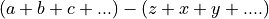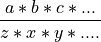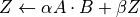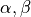# Optimizations¶

Aesara applies many kinds of graph rewrites, some of which can be considered “optimizations”:

The optimizations are listed in roughly chronological order. The table below gives a quick summary of the optimizations included in the default modes. The descriptions are brief and point to further reading.

If you would like to add an additional optimization, see Graph Rewriting.

When compiling, we can make a tradeoff between compile-time and run-time. Faster compile times will result in fewer optimizations being applied, hence generally slower run-times. For making this tradeoff when compiling, we provide a set of 4 optimization modes, ‘o1’ to ‘o4’, where ‘o1’ leads to fastest compile-time and ‘o4’ leads to fastest run-time in general. For an even faster run-time, we could disable assertions (which could be time consuming) for valid user inputs, using the optimization mode ‘unsafe’, but this is, as the name suggests, unsafe. See Unsafe Rewrites.

Note

This list is partial.

The print_summary method allows several OpDBs and optimizers to list the executed optimizations. This makes it possible to have an up-to-date list.

python -c "import aesara; aesara.compile.optdb.query(aesara.compile.predefined_optimizers['<OPT_ID>']).print_summary()"


where <OPT_ID> can be one of o1 (), o2, o3, o4 (*), Stabilization or unsafe.

Optimization o4 * o3 o2 o1 Stabilization unsafe
merge x x x x   x
constant folding x x x x   x
shape promotion x x       x
fill cut x x       x
inc_subtensor srlz. x x       x
reshape_chain x x       x
const. elimination x x       x
mul canonical. x x       x
dot22 x x       x
sparse_dot x x       x
sum_scalar_mul x x       x
neg_neg x x       x
neg_div_neg x x       x
mul specialize x x       x
pow specialize x x       x
inplace_setsubtensor x
gemm x x       x
inplace_elemwise x
inplace_random x
elemwise fusion x x x     x
local_log_softmax x x     x x
local_remove_all_assert           x

Note

*) o4 is equivalent to fast_run

†) o1 is equivalent to fast_compile

merge

A simple optimization in which redundant Apply nodes are combined. For example, in function([x,y], [(x+y)*2, (x+y)*3]) the merge optimization will ensure that x and y are only added once.

This optimization is very useful because it frees users to write highly redundant mathematical code. Aesara will make sure to compute just what is necessary.

See MergeOptimizer.

constant folding

When all the inputs to an expression are constant, then the expression can be pre-computed at compile-time.

See opt.constant_folding()

shape promotion

Aesara often knows how to infer the shape of an output from the shape of its inputs. Without this optimization, it would otherwise have to compute things (e.g. log(x)) just to find out the shape of it!

See opt.local_shape_lift_*()

fill cut

Fill(a,b) means to make a tensor of the shape of a full of the value b. Often when fills are used with elementwise operations (e.g. f) they are un-necessary: * f(fill(a,b), c) -> f(b, c) * f(fill(a, b), fill(c, d), e) -> fill(a, fill(c, f(b, d, e)))

See opt.local_fill_sink()

inc_subtensor serialization

Incrementing a small subregion of a large tensor can be done quickly using an inplace operation, but if two increments are being done on the same large tensor, then only one of them can be done inplace. This optimization reorders such graphs so that all increments can be done inplace.

inc_subtensor(a,b,idx) + inc_subtensor(a,c,idx) -> inc_subtensor(inc_subtensor(a,b,idx),c,idx)

See local_IncSubtensor_serialize()

reshape_chain

This optimizes graphs like reshape(reshape(x, shape1), shape2) -> reshape(x, shape2)

See local_reshape_chain()

constant elimination

Many constants indicate special cases, such as pow(x,1) -> x. Aesara recognizes many of these special cases.

See local_mul_specialize(), local_mul_specialize(),:func:local_mul_specialize

Rearrange expressions of additions and subtractions to a canonical form:See AlgebraicCanonizer, local_add_canonizer

mul canonicalization

Rearrange expressions of multiplication and division to a canonical form:See AlgebraicCanonizer, local_mul_canonizer

dot22

This simple optimization replaces dot(matrix, matrix) with a special dot22 op that only works for matrix multiplication. This op is implemented with a call to GEMM, and sometimes replaced entirely by the gemm optimization.

See local_dot_to_dot22()

sparse_dot

Aesara has a sparse matrix multiplication algorithm that is faster in many cases than scipy’s (for dense matrix output). This optimization swaps scipy’s algorithm for ours.

See local_structured_dot()

sum_scalar_mul

This optimizes graphs like sum(scalar * tensor) -> scalar * sum(tensor)

See local_sum_mul_by_scalar()

neg_neg

Composition of two negatives can be cancelled out.

See local_neg_neg()

neg_div_neg

Matching negatives in both the numerator and denominator can both be removed.

See local_neg_div_neg()

This optimization simplifies expressions involving the addition of zero.

See local_add_specialize()

mul specialization

Several special cases of mul() exist, and this optimization tries to recognize them. Some examples include: * mul(x,x) -> x**2 * mul(x,0) -> zeros_like(x) * mul(x, -1) -> neg(x)

See local_mul_specialize()

pow specialization

Several special cases of pow() exist, and this optimization tries to recognize them. Some examples include: * pow(x,2) -> x**2 * pow(x,0) -> ones_like(x) * pow(x, -0.5) -> reciprocal(sqrt(x))

See local_pow_specialize()

inplace_setsubtensor

In order to be a pure Op, setsubtensor must copy its entire input, and modify just the subtensor in question (possibly a single element). It is much more efficient to modify that element inplace.

See local_inplace_setsubtensor()

gemm

Numerical libraries such as MKL and ATLAS implement the BLAS-level-3 interface, and provide a function GEMM that implements, for matrices A, B and Z, and scalars.

This optimization tries to rearrange a variety of linear algebra expressions into one or more instances of this motif, and replace them each with a single Gemm Op.

See GemmOptimizer

inplace_elemwise

When one of the inputs to an elementwise expression has the same type and shape as the output, and is no longer needed for computation after the elemwise expression is evaluated, then we can reuse the storage of the input to store the output.

See insert_inplace_optimizer()

inplace_random

Typically when a graph uses random numbers, the RandomState is stored in a shared variable, used once per call and, updated after each function call. In this common case, it makes sense to update the random number generator in-place.

See random_make_inplace()

elemwise fusion

This optimization compresses subgraphs of computationally cheap elementwise operations into a single Op that does the whole job in a single pass over the inputs (like loop fusion). This is a win when transfer from main memory to the CPU is a bottleneck.

See FusionOptimizer

local_log_softmax
This is a stabilization optimization. It can happen due to rounding errors that the softmax probability of one value gets to 0. Taking the log of 0 would generate -inf that will probably generate NaN later. We return a closer answer.
local_remove_all_assert

This is an unsafe optimization. For the fastest possible Aesara, this optimization can be enabled by setting optimizer_including=local_remove_all_assert which will remove all assertions in the graph for checking user inputs are valid. Use this optimization if you are sure everything is valid in your graph.